## ML Aggarwal Class 7 Solutions for ICSE Maths Chapter 7 Percentage and Its applications Ex 7.3

Questions 1.
Rohan bought a calculator for ₹ 760 and sold it for ₹ 874. Find his profit and profit percentage.
Solution:
C.P. of calculate = ₹ 760
and S.P. = ₹ 874
Gain = S.P. – C.P. = ₹ 874 – ₹ 760 = ₹ 114Question 2.
Kirti bought a saree for ₹ 2500 and sold it for ₹ 2300. Find her loss and loss percent.
Solution:
C.P. of a saree = ₹ 2500
and S.P. = ₹ 2300
Loss = C.P. – S.P = ₹ 2500 – ₹ 2300 = ₹ 200Question 3.
Tell what is profit or loss in the following transactions. Also find profit percent or loss percent in each case:
(i) Gardening shears bought for ₹ 250 and sold for ₹ 325.
(ii) A shirt bought for ₹ 250 and sold at ₹ 150.
Solution:
(i) C.P. of gardening shears = ₹ 250 and S.P. = ₹ 325
Gain = S.P. – C.P. = ₹ 325 – ₹ 250 = ₹ 75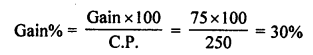(ii) C.P. of a shirt = ₹ 250 and S.P. = ₹ 150
Loss = C.P. – S.P. = ₹ 250 – ₹ 150 = ₹ 100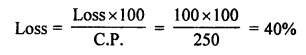Question 4.
Rajinder bought one almirah for ₹ 4800 and the other for ₹ 3640. He sold the first almirah at a gain of 13$$\frac { 1 }{ 3 }$$ % and the other at a loss of 15%. How much did he gain or lose in the whole deal?
Solution:
C.P. of one almirah = ₹ 4800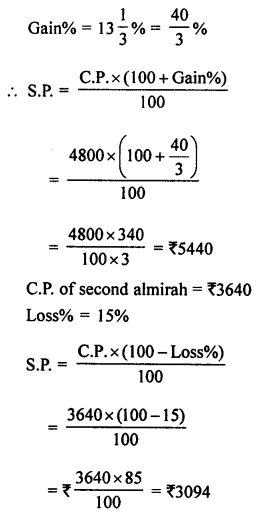C.P. of both the almirahs = ₹ 4800 + ₹ 3640 = ₹ 8440
and S.P. = ₹ 5440 + ₹ 3094 = ₹ 8534
Total gain = S.P. – C.P. = ₹ 8534 – ₹ 8440 = ₹ 94

Question 5.
In a furniture shop, 24 tables were bought at the rate of ₹ 450 per table. The shopkeeper sold 16 of them at the rate of ₹ 600 per table and the remaining at the rate of ₹ 400 per table. Find his gain or loss percent.
Solution:
Price of one table = ₹ 450
C.P. of 24 tables = ₹ 450 × 24 = ₹ 10800
S.P. of 16 tables at the rate of ₹ 600 = ₹ 600 × 16 = ₹ 9600
S.P. of remaining (24 – 16) = 8 tables = ₹ 400 × 8 = ₹ 3200
Total S.P. = ₹ 10800 + ₹ 3200 = ₹ 14400
Gain = S.P. – C.P. = ₹ 14400 – ₹ 10800 = ₹ 3600Question 6.
By selling a fan for ₹ 810, a dealer makes a profit of ₹ 60. What is the cost price of the fan? What is his profit percent?
Solution:
S.P. of a fan = ₹ 810
Profit = ₹ 60
Cost price = S.P. – Profit = ₹ 810 – ₹ 60 = ₹ 750Question 7.
By selling a steel almirah for ₹ 3906, a manufacturer suffers a loss of ₹ 294. Find the cost price of the almirah and his loss percentage.
Solution:
S.P. of a steel almirah = ₹ 3906
Loss = ₹ 294
C.P. = S.P. + Loss = ₹ 3906 + ₹ 294 = ₹ 4200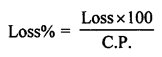Question 8.
The cost price of a flower vase is ₹ 120. If the shopkeeper sells it at a loss of 10%, find the price at which it was sold.
Solution:
C.P. of a flower vase = ₹ 120
Loss = 10%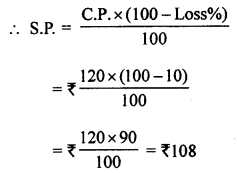Question 9.
I buy a T.V. for ₹ 10000 and sell it at a profit of 20%. How much money do I get for it?
Solution:
C.P. of a T.V. = ₹ 10000
Profit = 20%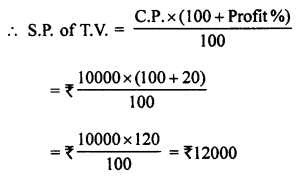Question 10.
A shopkeeper sells an article at ₹ 300, thus earning a profit of 20%. Find the cost price of the article.
Solution:
S.P. of an article = ₹ 300
Profit = 20%Question 11.
A shopkeeper sells i n article at ₹ 320, thus suffering a loss of 20%. Find the cost price of the article.
Solution:
S.P. of an article = ₹ 320
Loss = 20%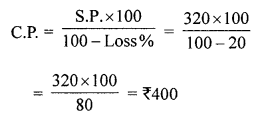Question 12.
By selling a chair for ₹ 522, a shopkeeper makes a profit of 16%. What is its cost price?
Solution:
S.P. of a chair = ₹ 522
Profit = 16%Question 13.
A trader sold some damaged garments for ₹ 7360 at a loss of 8%. Find the cost price of the garments.
Solution:
S.P. of damaged garments = ₹ 7360
Loss = 8%Question 14.
By selling a table for ₹ 3168, Rashid loses 12%. Find its cost price. What percent would he gain or lose by selling the table for ₹ 3870?
Solution:
S.P. of a table = ₹ 3168
Loss = 12%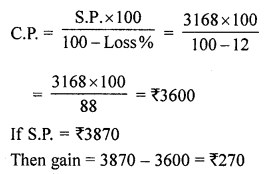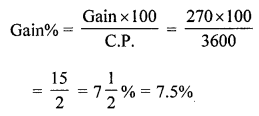Question 15.
By selling an article for ₹ 4550, Tony incurs a loss of 9%. What percent would he gain or lose by selling it for ₹ 4825?
Solution:
S.P. of an article = ₹ 4550
Loss = 9%